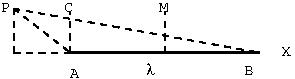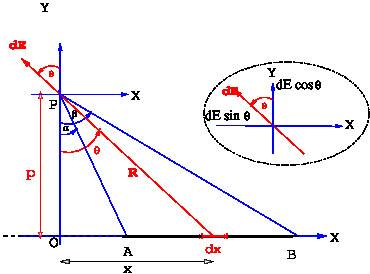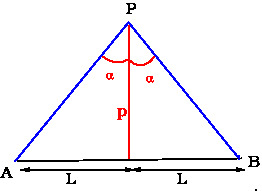# [YMP/EM-02004] Electric Field due to a Line with Uniform Charge Density

For page specific messages
For page author info

Let $$AB$$ be a line charge with charge per unit length $\lambda$, which is given to be uniform. The field is to be computed at a point $$P$$,. Let $$p$$ be the perpendicular distance of $$P$$ from the line $$AB$$. let $$\alpha, \beta$$ be the angles as shown in the figure. Electric field will be calculated at a general point $$P$$, the corner point $$C$$ and the middle point $$M$$ as shown in figure below.Let $$AB$$ be a line charge with charge per unit length $\lambda$, which is given to be uniform. The field is to be computed at a point $$P$$, see \Figref{LineCharge}. Let $$p$$ be the perpendicular distance of $$P$$ from the line \  angles as shown in the figure. Electric field is  calculated at different points $$P$$ as shown in figures.Field at a general point

We need to choose a coordinate system. Let $$X$$- axis be  taken along the line charge, the $$Y$$- axis be chosen to pass through the point $$P$$.
We need to separately compute the $$x$$- and $$y$$- components of the electric field due to an element $$dx$$ at position $$x$$ and integrate over $$x$$. The field components  due  to the small line element $$dx$$ are\begin{eqnarray} {E}_x&=& \frac{\lambda dx}{4\pi\epsilon_0R^2}(-\sin \theta) \label{EQ01}\\
{E}_y&=&\frac{\lambda dx}{4\pi\epsilon_0R^2}(\cos\theta) \label{eq2} \end{eqnarray}  The electric field is obtained by integrating over $$x$$.
\begin{eqnarray} {E}_x&=&\int\frac{\lambda dx}{4\pi\epsilon_0R^2}(-\sin \theta) \label{EQ02} \\{E}_y&=&\int\frac{\lambda dx}{4\pi\epsilon_0R^2}(\cos\theta) \label{eq2a} \end{eqnarray} It is convenient to change the integration variable from $$x$$ to $$\theta$$ defined by $x=p \tan\theta$, and $dx=p\sec^2\theta d\theta$, with $R=p\sec\theta$, we get \begin{eqnarray}
\nonumber {E}_x&=&\int_{\alpha}^{\beta}\frac{\lambda
p\sec^2\theta}{(4\pi\epsilon_0)p^2\sec^2\theta}(-\sin \theta)d\theta\\
\nonumber&=&\frac{\lambda}{4\pi\epsilon_0\,p}
\int_{\alpha}^{\beta}(-\sin \theta)d\theta\\
{E}_x&=&\frac{\lambda}{4\pi\epsilon_0\,p} (\cos\beta - \cos\alpha)
\label{eq3}
\end{eqnarray} Similarly, the $$y$$- component of the field works out to be
\begin{eqnarray} {E}_y&=&-\frac{\lambda}{4\pi\epsilon_0p} (\sin \beta - \sin \alpha) \label{eq4} \end{eqnarray} Thus \begin{equation} \vec E = \frac{\lambda}{4\pi\epsilon_0}\big\{(\cos\beta-\cos \alpha) \hat i + (\sin \alpha -\sin\beta) \hat j\big\} \end{equation}

Field at corner  point

For a point above a corner $$\alpha=0,\beta=\pi/2$$ and the field is given by

$E_y= - \frac{\lambda}{4\pi\epsilon_0 p}$

Field at a point on the bisectorHere $\beta=-\alpha$ and $\sin \alpha=\frac{L}{\sqrt{L^2+p^2}}$ . Therefore \begin{eqnarray} {E}_y&=&\frac{\lambda }{2\pi\epsilon_0\,p}\frac{L}{\sqrt{L^2+p^2}}
\label{eq5}\\{E}_x&=&0 \label{eq6}\end{eqnarray}

Field due to an infinite line charge
In this case $$\alpha=-\pi/2. \beta=\pi/2$$. Therefore the electric field at a distance $$p$$ is \begin{equation} E_y = \frac{\lambda}{2\pi\epsilon_0 p}. \end{equation}  This agrees with $$L\to \infty$$ limit of \eqref{eq5}. In the above treatment, the field has been computed at points on the $$Y$$- axis. However, due to the rotational symmetry about the $$X$$- axis, the value of electric field will be same at all points in the $$Y-Z$$ plane at a fixed distance $$\rho$$ from on the $$X$$-axis.

n
0

X